Next: Inverse-Square Force Law Up: Some Consequences Previous: Tidal Forces, Gravitational Waves

### Gravitational Collapse

Another remarkable feature of Einstein's equation is the pressure term: it says that not only energy density but also pressure causes gravitational attraction. This may seem to violate our intuition that pressure makes matter want to expand! Here, however, we are talking about gravitational effects of pressure, which are undetectably small in everyday circumstances. To see this, let's restore the factors of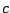. Also, let's remember that in ordinary circumstances most of the energy is in the form of rest energy, so we can write the energy densityas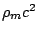, where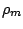is the ordinary mass density: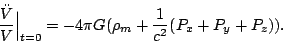On the human scale all of the terms on the right are small, sinceis very small. (Gravity is a weak force!) Furthermore, the pressure terms are much smaller than the mass density term, since they are divided by an extra factor of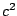.

There are a number of important situations in whichdoes not dominate over. In a neutron star, for example, which is held up by the degeneracy pressure of the neutronium it consists of, pressure and energy density contribute comparably to the right-hand side of Einstein's equation. Moreover, above a mass of about 2 solar masses a nonrotating neutron star will inevitably collapse to form a black hole, thanks in part to the gravitational attraction caused by pressure. In fact, any object of mass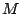will form a black hole if it is compressed to a radius smaller than its Schwarzschild radius,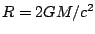.

Next: Inverse-Square Force Law Up: Some Consequences Previous: Tidal Forces, Gravitational Waves

© 2006 John Baez and Emory Bunn